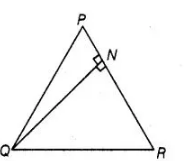# In a Δ PQR, N is a point on PR,

Question:

In a Δ PQR, N is a point on PR, such that QN ⊥ PR. If PN . NR = QN2, then prove that ∠PQR = 90°.

Solution:

Given ΔPQR, N is a point on PR, such that QN ⊥ PRand $P N \cdot N R=Q N^{2}$

To prove $\angle P Q R=90^{\circ}$

$\begin{array}{ll}\text { Proof We have, } & P N \cdot N R=Q N^{2}\end{array}$

$\Rightarrow \quad P N \cdot N R=Q N \cdot Q N$

$\Rightarrow \quad \frac{P N}{Q N}=\frac{Q N}{N R} \quad \ldots$ (i)

In $\triangle Q N P$ and $\triangle R N Q$, $\frac{P N}{Q N}=\frac{Q N}{N R}$

and $\angle P N Q=\angle R N Q$ [each equal to $90^{\circ}$ ]

$\therefore$ $\triangle Q N P \sim \triangle R N Q$ [by SAS similarity criterion]

Then, $\triangle Q N P$ and $\Delta R N O$ are equiangulars.

i.e., $\angle P Q N=\angle Q R N$

$\angle R Q N=\angle Q P N$

On adding both sides, we get

$\angle P Q N+\angle R Q N=\angle Q R N+\angle Q P N$

$\Rightarrow$ $\angle P Q R=\angle Q R N+\angle Q P N$ ....(ii)

We know that, sum of angles of a triangle $=180^{\circ}$

In $\triangle P Q R_{1} \quad \angle P Q R+\angle Q P R+\angle Q R P=180^{\circ}$

$\Rightarrow \quad \angle P Q R+\angle Q P N+\angle Q R N=180^{\circ} \quad[\because \angle Q P R=\angle Q P N$ and $\angle Q R P=\angle Q R M]$

$\Rightarrow \quad \angle P Q R+\angle P Q R=180^{\circ} \quad$ [using Eq. (ii)]

$\Rightarrow \quad 2 \angle P Q R=180^{\circ}$

$\Rightarrow \quad \angle P Q R=\frac{180^{\circ}}{2}=90^{\circ}$

$\therefore \quad \angle P Q R=90^{\circ}$

Hence proved.